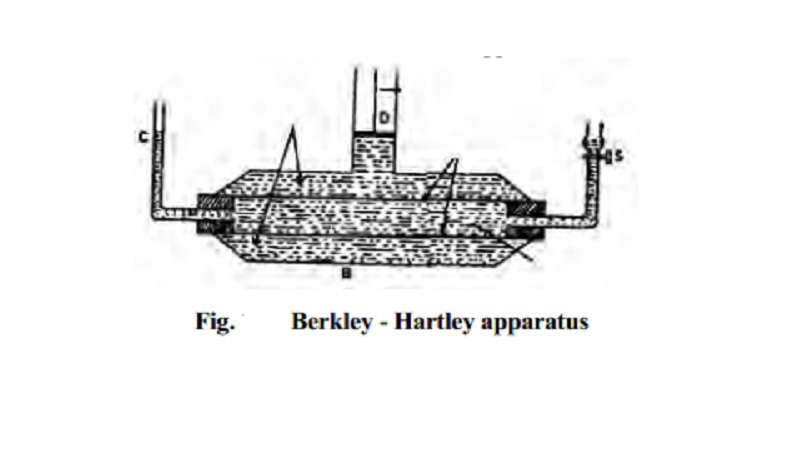Home | | Chemistry | Determination of molecular weight and osmotic pressure by Berkley-Hartley method

# Determination of molecular weight and osmotic pressure by Berkley-Hartley method1.Boyle's - Vant Hoff law The osmotic pressure (Pi) of the solution at temperature is directly propositional to the concentration (C) of the solution. 2. Charle's - Vant Hoff law At constant concentration the osmotic pressure(Pi) is directly proportional to the temperature (T).

Osmotic pressure and concerned laws

Vant Hoff noted the striking resemblance between the behaviour of dilute solutions and gases. He concluded that, a substance in solution behaves exactly like gas and the osmotic pressure of a dilute solution is equal to the pressure which the solute would exert if it is a gas at the same temperature occupying the same volume as the solution. Thus it is proposed that solutions also obey laws similar to gas laws.

1.Boyle's - Vant Hoff law

The osmotic pressure (Pi) of the solution at temperature is directly propositional to the concentration (C) of the solution.

Pi Dir. Props to C at constant T

C = Molar concentration

2. Charle's - Vant Hoff law

At constant concentration the osmotic pressure(Pi) is directly proportional to the temperature (T).

Pi dirc.Prop.to T at constnt C.

Combining these two laws,

Pi = CRT

Where R is the gas constant.

Determination of molecular weight by osmotic pressure measurement

The osmotic pressure is a colligative property as it depends, on the number of solute molecules and not on their identity.

Solution of known concentration is prepared by dissolving a known weight (W2) of solute, in a known volume (V dm3) of the solvent and its osmotic pressure is measured at room temperature(T).

Since Pi = CRT

C = n2/ V = number of moles of solute / Volume of the solution in dm3

C= W2 / M2V

We get

Pi = W2 R T  / M2V

M2 = W2 R T  / Pi V

Thus M2, molecular weight of the solute can be calculated by measuring osmotic pressure value.

Determination of osmotic pressure by Berkley-Hartley method

The osmotic pressure of a solution can be conveniently measured by Berkley - Hartley method. The apparatus (Fig.) consists of two concentric tubes. The inner tube (a) is made of semipermeable membrane(c) with two side tubes. The outer tube (b) is made of gun metal which contains the solution. The solvent is taken in the inner tube. As a result of osmosis, there is fall of level in the capillary indicator (d) attached to the inner tube. The external pressure is applied by means of a piston (e) attached to the outer tube so that the level in the capillary indicator remains stationary at (d). This pressure is equal to the osmotic pressure (p) and the solvent flow from inner to outer tube is also stopped.

The osmotic pressure is recorded directly and the method is quick.

There is no change in the concentration of the solution during the measurement of osmotic pressure.

The osmotic pressure is balanced by the external pressure and there is minimum strain on the semipermeable membrane.Study Material, Lecturing Notes, Assignment, Reference, Wiki description explanation, brief detail
11th 12th std standard Class Organic Inorganic Physical Chemistry Higher secondary school College Notes : Determination of molecular weight and osmotic pressure by Berkley-Hartley method |